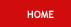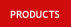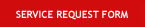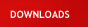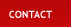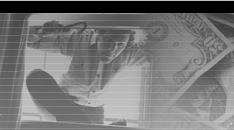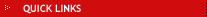Monday, June 05, 2023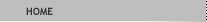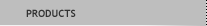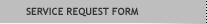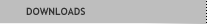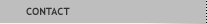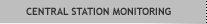Amount: \$ USDMust fill in amount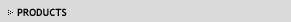Products Section Text goes here and things and such. About the things that go here. Here we have the things that go. The go things are that be here. This is here where go things belong. Text goes here and things and such. About the things that go here. Here we have the things that go. The go things are that be here. This is here where go things belong. Text goes here and things and such. About the things that go here. Here we have the things that go. The go things are that be here. This is here where go things belong. Text goes here and things and such. About the things that go here. Here we have the things that go. The go things are that be here. This is here where go things belong. Text goes here and things and such. About the things that go here. Here we have the things that go. The go things are that be here. This is here where go things belong. Additional Points of Interest Here we have additional points of interest. Additional Points of Interest Here we have additional points of interest.